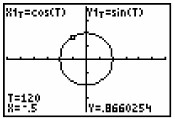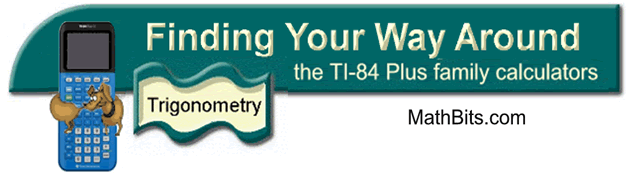Unit Circle Investigation

Listed below are two investigations that will allow you to investigate the workings of the unit circle and the relationship between the unit circle and the trigonometric graphs.

 Animated display of the relationship between the unit circle and the creation of the trigonometric curves Set the MODE to Radian, Parametric (Par), and Simultaneous (Simul).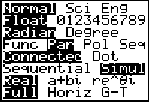Set the WINDOW to: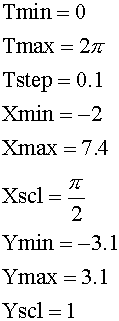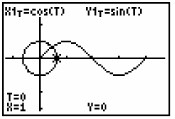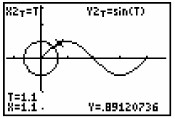Use the up and down arrows to  move between the unit circle and the trig curve. Enter the equations: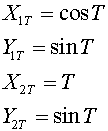Press GRAPH.  The Unit Circle and the Trigonometric graph will be drawn simultaneously.  Trace between the unit circle and the trig curve to see the relationships between the graphs. A further exploration can be demonstrated by changing Y2T = cos T  and again tracing between the graphs.

 Investigate the Unit Circle more closely: Set the MODE to Degree, Parametric (Par), Sequential and clear the equations.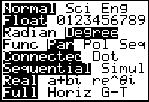Set the WINDOW to: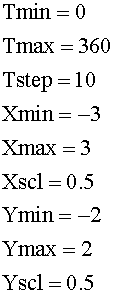Note:  The Tstep controls the increment of angle rotation.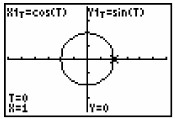Enter the equations: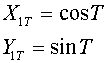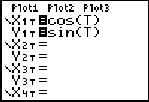Press TRACE and arrow to the right.  The spider will work its way around the unit circle examining the angles as it progresses.